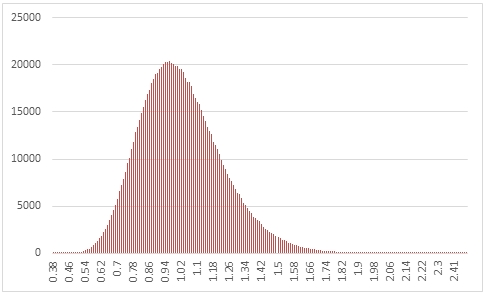# SQL Server random F-distribution

RANDFDIST

Updated: 31 March 2014

Use the table-valued function RANDFDIST to generate a sequence of random numbers from an F-distribution with the degree of freedom parameters @df1 and @df2.
Syntax
SELECT * FROM [wctMath].[wct].[RANDFDIST](
<@Rows, int,>
,<@df1, float,>
,<@df2, float,>)
Arguments
@Rows
the number of rows to generate. @Rows must be of the type int or of a type that implicitly converts to int.
@df1
the first degree of freedom parameter. @df1 must be of the type float or of a type that implicitly converts to float.
@df2
the second degree of freedom parameter. @df2 must be of the type float or of a type that implicitly converts to float.
Return Types
RETURNS TABLE (
[Seq] [int] NULL,
[X] [float] NULL
)
Remarks
·         @df1 must be greater than zero.
·         @df2 must be greater than zero.
·         If @df1 is NULL then @df1 is set to 1.
·         If @df2 is NULL then @df2 is set to 1.
·         If @Rows is less than 1 then no rows are returned.
Examples
In this example we create a sequence 1,000,000 random numbers rounded to two decimal places from an F-distribution with @df1 = 100 and @df2 = 100, COUNT the results, paste then into Excel and graph them.
SELECT
X,
COUNT(*) as [COUNT]
FROM (
SELECT
ROUND(X,1) as X
FROM
wct.RANDFDIST(
1000000, --@Rows
100,     --@df1
100      --@df2
)
)n
GROUP BY
X
ORDER BY
X

This produces the following result.In this example we generate 1,000,000 random numbers from an F-distribution with @df1 of 100 and a @df2 of 100. We calculate the mean, standard deviation, skewness, and excess kurtosis from the resultant table and compare those values to the expected values for the distribution.
DECLARE @size as int = 1000000
DECLARE @d1 as float = 100
DECLARE @d2 as float = 100
DECLARE @mean as float =
CASE
WHEN @d2 <= 2 THEN NULL
ELSE @d2/(@d2-2)
END
DECLARE @var as float =
CASE
WHEN @d2 <=4 THEN NULL
ELSE (2*POWER(@d2,2)*(@d1+@d2-2))/(@d1*POWER(@d2-2,2)*(@d2-4)) END
DECLARE @stdev as float =
CASE
WHEN @d2 <=4 THEN NULL
ELSE SQRT(@var) END
DECLARE @skew as float =
CASE
WHEN @d2 <= 6 THEN NULL
ELSE ((2*@d1+@d2-2)*SQRT(8*(@d2-4)))/((@d2-6)*SQRT(@d1*(@d1+@d2-2)))
END
DECLARE @kurt as float =
CASE
WHEN @d2 <= 8 THEN NULL
ELSE 12*((@d1*(5*@d2-22)*(@d1+@d2-2)+(@d2-4)*POWER(@d2-2,2))/(@d1*(@d2-6)*(@d2-8)*(@d1+@d2-2)))
END

SELECT
stat,
[RANDFDIST],
[EXPECTED]
FROM (
SELECT
x.*
FROM (
SELECT
AVG(x) as mean_FDIST,
STDEVP(x) as stdev_FDIST,
wct.SKEWNESS_P(x) as skew_FDIST,
wct.KURTOSIS_P(x) as kurt_FDIST
FROM
wct.RANDFDIST(@size,@d1,@d2)
)n
CROSS APPLY(
VALUES
('RANDFDIST','avg', mean_FDIST),
('RANDFDIST','stdev', stdev_FDIST),
('RANDFDIST','skew', skew_FDIST),
('RANDFDIST','kurt', kurt_FDIST),
('EXPECTED','avg',@mean),
('EXPECTED','stdev',@stdev),
('EXPECTED','skew',@skew),
('EXPECTED','kurt',@kurt)
)x(fn_name,stat,val_stat)
)d
PIVOT(sum(val_stat) FOR fn_name in([RANDFDIST],[EXPECTED])) P

This produces the following result (your result will be different).

 stat RANDFDIST EXPECTED avg 1.020150551 1.020408163 kurt 0.694350268 0.727888319 skew 0.617036971 0.624361946 stdev 0.207013091 0.207245878

### SupportCopyright 2008-2023 Westclintech LLC         Privacy Policy        Terms of Service